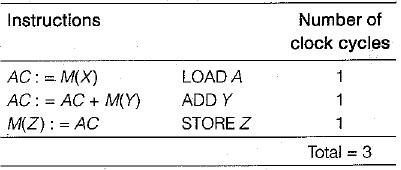Courses

## 15 Questions MCQ Test Question Bank for GATE Computer Science Engineering | Addressing Modes (Advance Level) - 1

Description
QUESTION: 1

### Consider a high-level language statement while [* i - -] then which addressing mode is suitable for it?

Solution:

In given while loop i is a pointer variable so autodecrement addressing mode is suitable.

QUESTION: 2

### Given below are some statements associated with the registers of a CPU. Identify the false statement.

Solution:

Program counter contain the address of next instruction to be fetched but not address of executed instruction.

QUESTION: 3

Solution:

Addressing modes are either explicitly specified or implied by the instruction.

QUESTION: 4

Which of the following is not valid class of interrupts?
1. Program
2. Timer
3. I/O
4. Hardware failure

Solution:

1, 2, 3, 4 are valid classes of interrupts:
1. Program: generated by some condition that occurs as a result of an instruction execution, such as arithmetic overflow, division by zero etc.
2. Timer: generated by a timer within the processor. This allows the operating system to perform certain functions on a regular basis.
3. I/O: generated by an I/O controller, to signal normal completion of an operation or to signal a variety of error condition.
4. Hardware failure: generated by failure such as power failure or memories parity error.

QUESTION: 5

System calls are usually invoked by using
1. An indirect jump
2. A software interrupt
3. Polling
4. A privileged instruction

Solution:

System call is a request in unit like operating system made via software interrupt by an active process for a service performed by the Kernal.

QUESTION: 6

Which of the following affects processing power?

Solution:

Processing power depends on clock speed, data bus capacity and addressing scheme.

QUESTION: 7

Microinstruction length is determined by _____.
1. The maximum number of simultaneous micro operations that must be specified.
2. The way in which the control information is represented or encoded.
3. The way in which the next microinstruction address is specified.

Solution:

Format of micro instruction: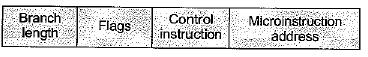QUESTION: 8

The following diagram shows, which addressing mode?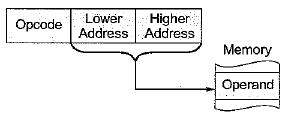Solution:

The diagram shows, extended addressing mode. In this, the effective memory address directly specified and it is used by some of the processor and address specified is 16 bit address.

QUESTION: 9

The word length of a CPU is defined as

Solution:

The word length of processor is defined as the width of a CPU registers i.e. at a time number of instruction process by the processor.

QUESTION: 10

In four-address instruction format, the number of bytes required to encode an instruction is (assume each address requires 24 bits, and 1 byte is required for operation code)

Solution: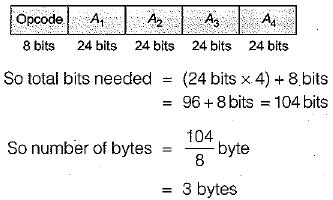QUESTION: 11

Match List-I with List-ll and select the correct answer using the codes given below the lists:
List-I
A. Stack overflow
B. Supervisor call
C. Invalid opcode
D. Tinner

List-ll
1. Software interrupt
2. Internal interrupt
3. External interrupt
4. Machine check interrupt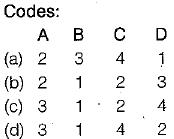Solution:

• Stack over flow is a internal interrupt.
• Supervisor call is a software interrupt.

• Invalid opcode is a internal interrupt.
• Timer is a external interrupt.

QUESTION: 12

Consider a CPU has 8 general-purpose registers
R0, R1..., R7 and supports the following operations.
ADD Ra, Rb, Rc Add Ra to Rb and store the result to Rc.
MUL Ra, Rb, Rc Multiply Ra to Rb and store the result to Rc.
An operation normally takes one clock cycles, an operation takes two clock cycles if it produces a result required by the immediately following operations. Consider the expression XY + XYZ + YZ, where variables X, Y and Z are initially located in the registers R0, R1 and R2. If contents these registers must not be modified, what is the minimum number of clock cycles required for an operation sequence that computes the value of XY + XYZ + YZ?

Solution: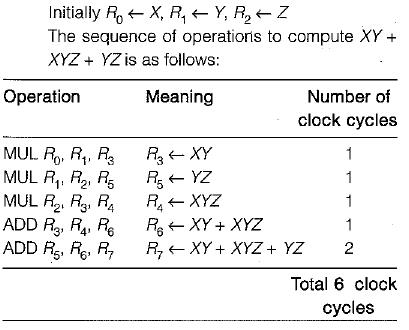QUESTION: 13

A CPU has an arithmetic unit that adds bytes and then sets its V, C and Z flag bits as follows. The V-bit is set if arithmetic overflow occurs (in 2’s complement arithmetic). The C-bit is set if a carry-out is generated from the most significant bit during an operation. The Z-bit is set if the result is zero. What are the values of the V, C and Z flag bit after 8-bit byte 1100 1100 and 10001111 are added?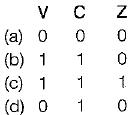Solution: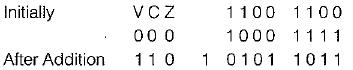V-bit is set to 1 due to arithmetic overflow.
C-bit is set to 1 because most significant digits generates a carry.
Z-bit is set to 0 because the result of addition is not zero.

QUESTION: 14

Consider the following sequence of instructions intended for execution on a stack machine. Each arithmetic operation pops the second operand, then pops the first operand, operates on them, and then pushes-the result back onto the stack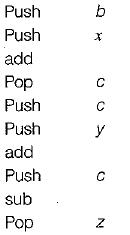Which of the following statements is/are true?
1. If push and pop instructions each require 5 bytes of storage, and arithmetic operations each require 1 byte of storage then the instruction sequence as a whole requires a total of 40 bytes of storage.
2. At the end of execution, zcontainsthe same value as y.
3. At the end of execution, the stack is empty.

Solution:

There are 7 pushes and pops for a cost of 35 bytes plus 3 arithmetic instructions for a total of 38 bytes storage.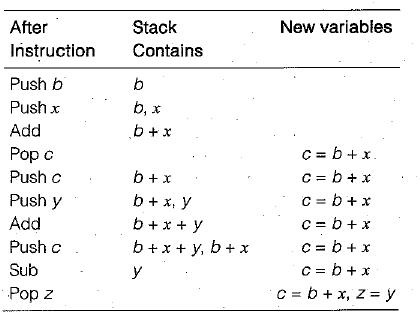QUESTION: 15

Consider an accumulator-based CPU supports only single address instruction. The CPU supports the following instructions: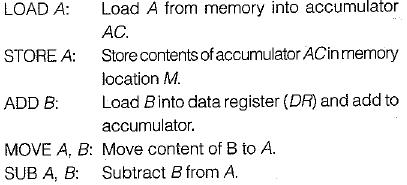Assume CPU uses the memory referencing and each instruction LOAD, STORE and ADD takes one clock cycle. To compute Z = X + Y CPU takes how many minimum number of clock cycles?

Solution: